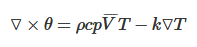# Solving equation to find an unknown function?

Hello,
I have simulated heat and fluid flow problem in Fluent and imported into Paraview.
I have the data for velocity, temperature fields.
Now I want to obtain an unknown function by solving the below equation:where the terms on the right hand side (density, specific heat, velocity vector, temperature, thermal conductivity) are known from data. The left hand side contains curl of an unknown function “Theta”. I want to find this “Theta” variation which gives me heat function distribution.
Is it possible? If so, I request the procedure to be done.

Thanks & Regards,

This is not something that is present in ParaView, nor in VTK I think.

You may be able to implement in a Programmable Filter.

Hello Mathieu,

Programmable filters like Python Calculator that is available in Paraview or some thing else?

Thanks,

Python Calculator and Programmable Filter are different things.

I do not think you can solve this in a python calculator.
Programmable Filter are similar but more complex :
https://www.paraview.org/Wiki/Python_Programmable_Filter

1 Like

If I understand your equation correctly, you first need the gradient of the temperature field. You can estimate that using either the `Gradient` or `Graident of Unstructured DataSet` filters.

The rest of the right side of the equation can be computed with a simple expression in the `Calculator` filter. That will give you the curl of theta.

I don’t think there is any way to compute theta from its curl. It is not in general possible to take the inverse of a curl (c.f. https://www.quora.com/What-is-the-inverse-operation-of-curl-and-divergence).